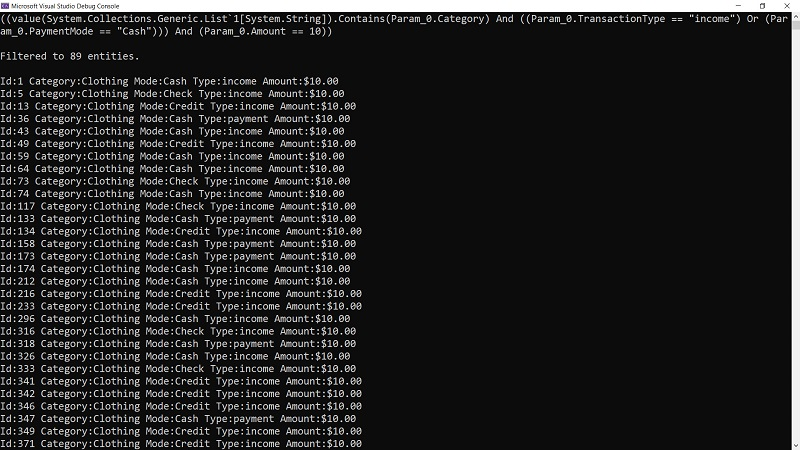# 深入 LINQ - 动态构建 LINQ 表达式

LINQ 是 Language Integrated Query（语言集成查询）的缩写，是我最喜欢的 .NET 和 C# 技术之一。使用 LINQ，开发者可以直接在强类型代码中编写查询。LINQ 提供了一种标准的语言和语法，使不同的数据源的查询编码方法一致。

## 一些基础

``````using System;
using System.Linq;

public class Program
{
public static void Main()
{
var someNumbers = new int[]{4, 8, 15, 16, 23, 42};
var query =
where num > 10
orderby num descending
select num.ToString();
Console.WriteLine(string.Join('-', query.ToArray()));
// 42-23-16-15
}
}
``````

``````var secondQuery = someNumbers.Where(n => n > 10)
.OrderByDescending(n => n)
.Select(n => n.ToString());
``````

1. 设置一个数据源，称为提供者（provider），供查询时使用。例如，到目前为止的代码使用了内置的 `LINQ to Objects` 提供者。你的 EF Core 项目使用的是 EF Core 提供者，它映射到你的数据库。
2. 查询被定义并转变成一个表达式树(expression tree)，我将在稍后介绍。
3. 查询被执行，数据被返回。

``````var found = false;
foreach(var item in secondQuery.AsEnumerable())
{
if (item == "23")
{
found = true;
break;
}
}
``````

## 难题

``````{
"condition": "and",
"rules": [
{
"label": "Category",
"field": "Category",
"operator": "in",
"type": "string",
"value": ["Clothing"]
},
{
"condition": "or",
"rules": [
{
"label": "TransactionType",
"field": "TransactionType",
"operator": "equal",
"type": "boolean",
"value": "income"
},
{
"label": "PaymentMode",
"field": "PaymentMode",
"operator": "equal",
"type": "string",
"value": "Cash"
}
]
},
{
"label": "Amount",
"field": "Amount",
"operator": "equal",
"type": "number",
"value": 10
}
]
}
``````

## 动态表达式

``````https://github.com/JeremyLikness/ExpressionGenerator
``````

``````var jsonStr = File.ReadAllText("rules.json");
var jsonDocument = JsonDocument.Parse(jsonStr);
``````

``````                         /-----------AND-----------\
|                         |
/-AND-\                      |
Category IN ['Clothing']   Amount eq 10.0        /-OR-\
TransactionType EQ 'income'  PaymentMode EQ 'Cash'
``````

## 引入 Transaction

``````public class Transaction
{
public int Id { get; set; }
public string Category { get; set; }
public string TransactionType { get; set; }
public string PaymentMode { get; set; }
public decimal Amount { get; set; }
}
``````

## 参数表达式

``````public Func<T, bool> ParsePredicateOf<T>(JsonDocument doc)
{
var itemExpression = Expression.Parameter(typeof(T));
var conditions = ParseTree<T>(doc.RootElement, itemExpression);
}
``````

``````var query = ListOfThings.Where(t => t.Id > 2);
``````

`t =>` 是一个参数，代表列表中一个条目的类型。因此，我们为该类型创建一个参数。然后我们递归地遍历 JSON 节点来建立树。

## 逻辑表达式

``````private Expression ParseTree<T>(
JsonElement condition,
ParameterExpression parm)
{
Expression left = null;
var gate = condition.GetProperty(nameof(condition)).GetString();

JsonElement rules = condition.GetProperty(nameof(rules));

Binder binder = gate == And ? (Binder)Expression.And : Expression.Or;

Expression bind(Expression left, Expression right) =>
left == null ? right : binder(left, right);
``````

`gate` 变量是条件，即“and”或“or”。规则语句得到一个节点，是相关规则的列表。我们正在跟踪表达式的左边和右边。`Binder` 签名是二元表达式的简写，定义如下：

``````private delegate Expression Binder(Expression left, Expression right);
``````

`binder` 变量简单地设置了顶层表达式：`Expression.And``Expression.Or`。两者都使用左边和右边表达式来计算。

`bind` 函数更有趣一点。当我们遍历树时，我们需要建立各种节点。如果我们还没有创建一个表达式（`left``null`），我们就从创建的第一个表达式开始。如果我们有一个现有的表达式，我们就用这个表达式来合并两边的内容。

``````foreach (var rule in rules.EnumerateArray())
``````

## 属性表达式

``````string @operator = rule.GetProperty(nameof(@operator)).GetString();
string type = rule.GetProperty(nameof(type)).GetString();
string field = rule.GetProperty(nameof(field)).GetString();
JsonElement value = rule.GetProperty(nameof(value));
var property = Expression.Property(parm, field);
``````

``````var filter = new List<string> { "Clothing" };
Transactions.Where(t => filter.Contains(t.Category));
``````

## 常量和调用表达式

``````private readonly MethodInfo MethodContains = typeof(Enumerable).GetMethods(
BindingFlags.Static | BindingFlags.Public)
.Single(m => m.Name == nameof(Enumerable.Contains)
&& m.GetParameters().Length == 2);
``````

``````if (@operator == In)
{
var contains = MethodContains.MakeGenericMethod(typeof(string));
object val = value.EnumerateArray().Select(e => e.GetString())
.ToList();
var right = Expression.Call(
contains,
Expression.Constant(val),
property);
left = bind(left, right);
}
``````

• 要调用的方法（`contains`
• 要检查的参数的值（带有 `Clothing` 的列表，或者 `Expression.Constant(val)`
• 要对其进行检查的属性（`t.Category`）。

``````Transactions.Where(t => (new List<string> { "Clothing" }).Contains(t.Category));
``````

``````if (rule.TryGetProperty(nameof(condition), out JsonElement check))
{
var right = ParseTree<T>(rule, parm);
left = bind(left, right);
continue;
}
``````

``````Transactions.Where(t => (new List<string> { "Clothing" }).Contains(t.Category) && <something>);
``````

## 比较表达式

``````object val = (type == StringStr || type == BooleanStr) ?
(object)value.GetString() : value.GetDecimal();
var toCompare = Expression.Constant(val);
var right = Expression.Equal(property, toCompare);
left = bind(left, right);
``````

``````Transactions.Where(t => t.TransactionType == "income");
``````

``````Transactions.Where(t => t.TransactionType == "income" || t.PaymentMode == "Cash");
``````

``````(
(value(System.Collections.Generic.List`1[System.String]).Contains(Param_0.Category)
And (
(Param_0.TransactionType == "income")
Or
(Param_0.PaymentMode == "Cash"))
)
And
(Param_0.Amount == 10)
)
``````

## Lambda 表达式和编译

``````var conditions = ParseTree<T>(doc.RootElement, itemExpression);
if (conditions.CanReduce)
{
conditions = conditions.ReduceAndCheck();
}
var query = Expression.Lambda<Func<T, bool>>(conditions, itemExpression);
return query.Compile();
``````

``````var predicate = jsonExpressionParser
.ParsePredicateOf<Transaction>(jsonDocument);
var transactionList = Transaction.GetList(1000);
var filteredTransactions = transactionList.Where(predicate).ToList();
filteredTransactions.ForEach(Console.WriteLine);
``````## 从内存到数据库

``````public Func<T, bool> ParsePredicateOf<T>(JsonDocument doc)
{
var query = ParseExpressionOf<T>(doc);
return query.Compile();
}
``````

`ExpressionGenerator` 程序使用编译后的查询，`DatabaseTest` 使用原始的 lambda 表达式。它将其应用于一个本地的 SQLite 数据库，以演示 EF Core 是如何解析表达式的。在数据库中创建并插入 1000 条 Transaction 后，通过下面代码查询总数：

``````var count = await context.DbTransactions.CountAsync();
Console.WriteLine(quot;Verified insert count: {count}.");
``````

``````SELECT COUNT(*)
FROM "DbTransactions" AS "d"
``````

``````var parser = new JsonExpressionParser();
var predicate = parser.ParseExpressionOf<Transaction>(
JsonDocument.Parse(
var query = context.DbTransactions.Where(predicate)
.OrderBy(t => t.Id);
var results = await query.ToListAsync();
``````

``````SELECT "d"."Id", "d"."Amount", "d"."Category", "d"."PaymentMode", "d"."TransactionType"
FROM "DbTransactions" AS "d"
WHERE ("d"."Category" IN ('Clothing') &
((("d"."TransactionType" = 'income') AND "d"."TransactionType" IS NOT NULL) |
(("d"."PaymentMode" = 'Credit') AND "d"."PaymentMode" IS NOT NULL))) &
("d"."Amount" = '10.0')
ORDER BY "d"."Id"
``````

## 总结

LINQ 表达式是一个非常强大的工具，可以过滤和转换数据。我希望这个例子有助于理解表达式树是如何构建的。当然，解析表达式树感觉有点像魔术。Entity Framework Core 是如何在表达式树上行走以产生有意义的 SQL？我正在自己探索这个问题，并得到了 `ExpressionVisitor` 类的帮助。我将陆续发表更多关于这个问题的文章。